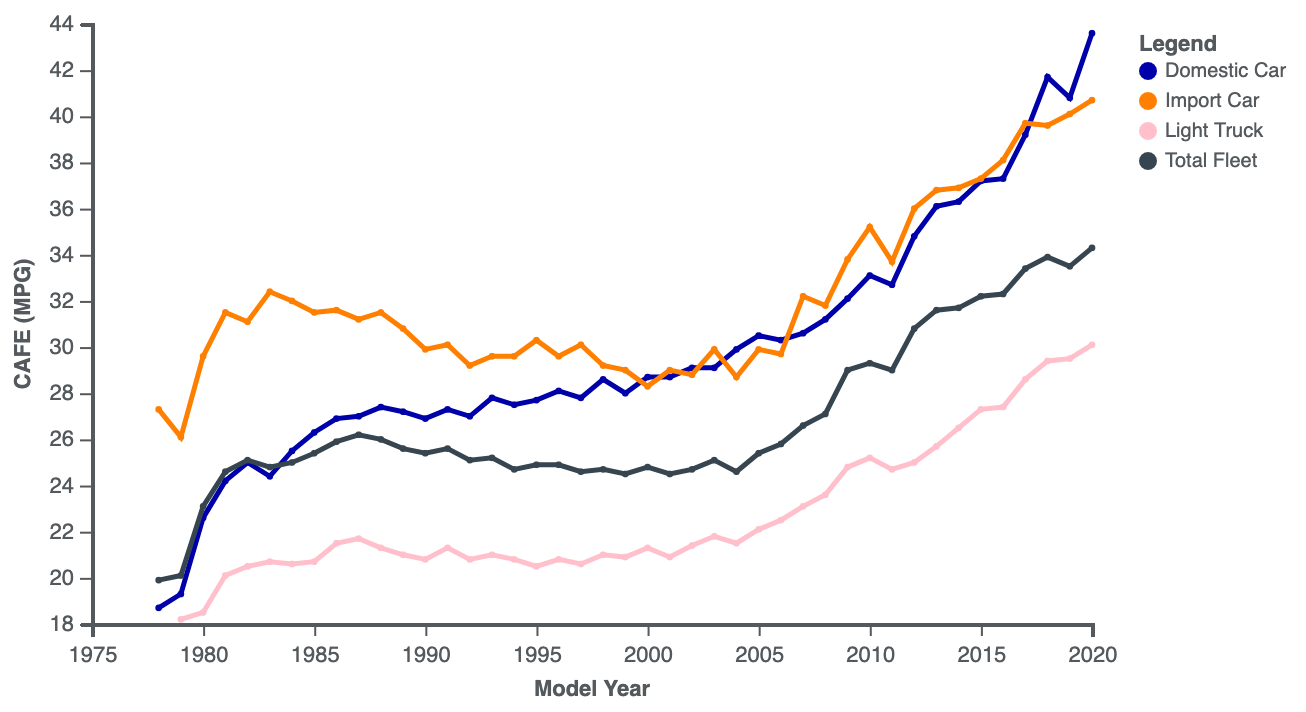# Calculator: kWh/100km to Liters/100km

## Conversion from kWh/100km to Liters/100km

With this algorithm, you can calculate the energy consumption of an electric vehicle, expressed in liters per 100 km, based on the data of consumption in kWh/100 km. This allows you to compare the energy efficiency of electric vehicles and vehicles with internal combustion engines on a single scale. The calculation is made through the MPGe ("Miles Per Gallon equivalent") indicator.

Enter the energy consumption of the electric vehicle in kWh per 100 km (miles):

The lower the energy consumption value, the more efficient your vehicle. Use the ARBA Auto apps to monitor the energy efficiency of your vehicle.

Calculation algorithm:

Converting kWh/100 km to miles per gallon equivalent (MPGe):

a. Convert 100 km to miles. 1 km is approximately 0.621371 miles. Thus, 100 km equals 100 * 0.621371 ≈ 62.1371 miles.

b. Calculate the energy consumption in kWh per 1 mile: (kWh/100 km) / 62.1371 miles.

c. Convert kWh/mile to MPGe, using the standard value for the USA: 33.705 MPGe/kWh. Multiply kWh/mile by 33.705 to get MPGe.

Converting MPGe to liters/100 km:

a. Considering that 1 MPGe is approximately equal to 1 mile per gallon (MPG), we can use the MPGe value for the following calculations.

b. Convert miles per gallon (MPG) to gallons per 100 miles: 100 / MPG.

c. Convert gallons per 100 miles to liters per 100 miles: (gallons per 100 miles) * 3.78541 (where 1 gallon ≈ 3.78541 liters).

d. Convert liters per 100 miles to liters per 100 km: (liters per 100 miles) / 1.60934 (where 1 mile ≈ 1.60934 km).

In the chart below, the average fuel consumption values in equivalent liters of gasoline / 100 km are shown for electric vehicles, hybrids, and internal combustion engine vehicles using various types of fuel.